# The MODEL Procedure

### Example 19.9 Circuit Estimation

Consider the nonlinear circuit shown in Output 19.9.1.

Output 19.9.1: Nonlinear Resistor Capacitor Circuit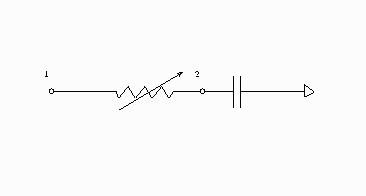The theory of electric circuits is governed by Kirchhoff’s laws: the sum of the currents flowing to a node is zero, and the net voltage drop around a closed loop is zero. In addition to Kirchhoff’s laws, there are relationships between the current I through each element and the voltage drop V across the elements. For the circuit in Output 19.9.1, the relationships are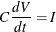for the capacitor and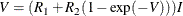for the nonlinear resistor. The following differential equation describes the current at node 2 as a function of time and voltage for this circuit: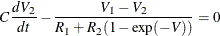This equation can be written in the form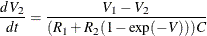Consider the following data.

data circ;
input v2 v1 time@@;
datalines;
-0.00007 0.0 0.0000000001 0.00912 0.5 0.0000000002
0.03091 1.0 0.0000000003 0.06419 1.5 0.0000000004
0.11019 2.0 0.0000000005 0.16398 2.5 0.0000000006
0.23048 3.0 0.0000000007 0.30529 3.5 0.0000000008
0.39394 4.0 0.0000000009 0.49121 4.5 0.0000000010
0.59476 5.0 0.0000000011 0.70285 5.0 0.0000000012
0.81315 5.0 0.0000000013 0.90929 5.0 0.0000000014
1.01412 5.0 0.0000000015 1.11386 5.0 0.0000000016
1.21106 5.0 0.0000000017 1.30237 5.0 0.0000000018
1.40461 5.0 0.0000000019 1.48624 5.0 0.0000000020
1.57894 5.0 0.0000000021 1.66471 5.0 0.0000000022
;


You can estimate the parameters in the preceding equation by using the following SAS statements:

title1 'Circuit Model Estimation Example';

proc model data=circ mintimestep=1.0e-23;
parm R2 2000  R1 4000 C 5.0e-13;
dert.v2 = (v1-v2)/((r1 + r2*(1-exp( -(v1-v2)))) * C);
fit v2;
run;


The results of the estimation are shown in Output 19.9.2.

Output 19.9.2: Circuit Estimation

 Circuit Model Estimation Example

The MODEL Procedure

Nonlinear OLS Parameter Estimates
Parameter Estimate Approx Std Err t Value Approx
Pr > |t|
R2 3002.471 1517.1 <------ Biased
R1 4984.842 1466.8 <------ Biased
C 5E-13 0 <------ Biased

 Note: The model was singular. Some estimates are marked 'Biased'.

In this case, the model equation is such that there is linear dependency that causes biased results and inflated variances. The Jacobian matrix is singular or nearly singular, but eliminating one of the parameters is not a solution in this case.